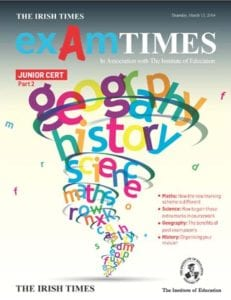# Exam Times – Junior Cert GeographyMichael Doran, who has been teaching Geography at The Institute of Education since 1995, explains how students can prepare effectively for this part of the exam…

There are a total of 150 marks awarded for the exam paper. There are two parts to the exam paper, Section 1 and Section 2.

#### SECTION 1 – 60 MARKS

There are twenty questions in this section. You have to answer all of the questions. If you do not answer a question, you will lose marks. Even if you are not sure of some of the answers, make sure that you at least try to answer each question. Section 1 accounts for 40% of the total exam mark.

• Each question is worth 3 marks.
• Each answer needs to be short, e.g., give a name.
• Some questions will provide multi-choice answers.
• Usually three or four questions will be about the Ordnance Survey map.
• You can allow up to forty-five minutes to answer Section 1. This then gives you at least two minutes to answer each question. Do not rush down your answer. Think carefully. Marks can be easily gained but they can be easily lost if you are careless.

NOTE – The Section 1 folder must be handed back with your answer book.

#### SECTION 2 – 90 MARKS

There are a total of five questions in Section 2. Each question is worth 30 marks. Students must choose three questions to answer. It is better to focus on answering three questions well rather than trying to answer four or five of the questions poorly.

• Each question is divided into three or four parts. If you pick a question, you have to answer each part of that question.
• At least one question will be about the Ordnance Survey map and the Aerial Photograph.
• One question is headed GEOGRAPHICAL MIX. This will include a number of different topics.
• You can allow up to 25 minutes answering each of the three questions.